# Inductance

## (7) The L-C circuit

• We already know that capacitance and inductor can store electrical and magnetic energy respectively
• when a charged capacitor is allowed to discharge through an resistance less inductor ,the current oscillates back and forth in the circuit
• Thus electrical oscillations of constant amplitude are produced in the circuit and are called L-C oscillations
• Let the capacitor of capacitance C be given a charge q0 and is then connected to an inductor as shown below in the figure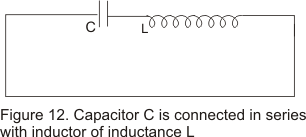• The moment the circuit is completed, charge on the capacitor begins to decrease giving rise to current in the circuit
• Suppose at any instant t during the discharge ,q is the amount of charge on the capacitor and I is the current through out the inductor
• EMF equation as obtained by Kirchhoff�s second law would be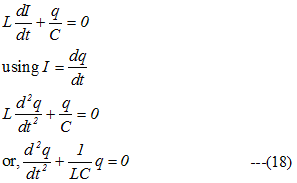• Comparing equation (18) with the SHM equation for mass spring system i.e.,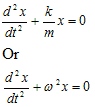where ω is the natural angular frequency of oscillations of undampled mass spring system, we can conclude that charge on the circuit oscillate with natural frequency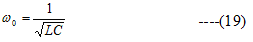and varies sinusoidal with time as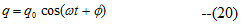where q0 is the maximum value of q and φ is the phase constant .
For initial phase φ=0,q=q0cosωt
• We thus see that LC circuit is identical to mass-spring system executing SHM
• Time period of oscillations is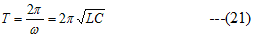and frequency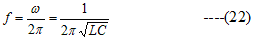• The current in the circuit is given by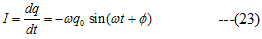Equation(23) indicates that the current in the circuit is also oscillatory and has the same frequency as charge
• In an L-C circuit ,during oscillations energy is partly electric and partly magnetic that is the oscillations consists of a transfer of energy back and forth from electric field of capacitor to magnetic field of the inductor
• The total energy of the circuit always remain constant and the situation is analogous to the transfer of energy in the mass-spring oscillation where energy alternates between two forms kinetic and potential
• Table given below compares the mechanic oscillations of mass spring system with that of electrical oscillations in an L-C circuit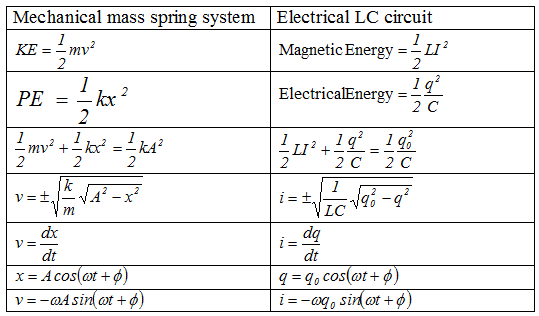## (8) Mutual Inductance

• Consider two coils 1 and 2 placed near each other as shown below in the figure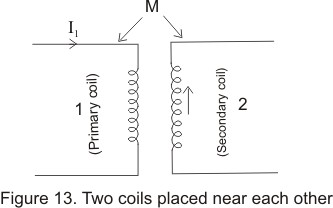• Let coil 1 be the primary coil and coil 2 be secondary coil
• When current is primary coil changes w.r.t time then the magnetic field produced in the coil also changes with time which causes a change in magnetic flux associated with secondary coil
• Due to this change of flux linked with secondary coil an emf is induced in it and this phenomenon is known as mutual induction
• Similarly change in current in secondary coil induces an emf in primary coild.This way as a result of mutual inductance emf is induced in both the coils
• If I1 is the current in primary coil at any instant ,than the emf induced in secondary coil would be proportional to the rate of change of current in primary coil i.e.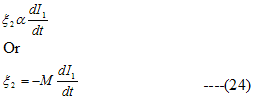Where M is a constant known as coefficient of mutual induction and minus sign indicates that direction of induced emf is such that it opposes the change of current in primary coil
• Unit of mutual inductance is Henry
• We know that a magnetic flux is produced in primary coil due to the flow of current I1.If this is the magnetic flux associated with secondary coil then from faraday's law of EM induction ,emf induced in secondary coil would be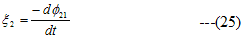• comparing equation (24) and (25) we get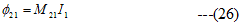Thus coefficient of mutual induction of secondary coil w.r.t primary coil is equal to magnetic flux linked with secondary coil when 1 Ampere of current flows in primary coil and vice-versa
• Similarly ,if I2 is the current in secondary coil at any instant then flux linked with primary coil is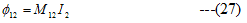where M12 is coefficient of mutual induction of primary coil with respect to secondary coil
• EMF induced in primary coil due to change of this flux is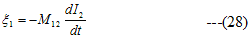• For any two circuits
M12=M21=M
• In general mutual inductance of two coil depends on geometry of the coils (shape ,size, number of turns etc),distance between the coils and nature of material on which the coil is wound

Note to our visitors :-

Thanks for visiting our website.
DISCLOSURE: THIS PAGE MAY CONTAIN AFFILIATE LINKS, MEANING I GET A COMMISSION IF YOU DECIDE TO MAKE A PURCHASE THROUGH MY LINKS, AT NO COST TO YOU. PLEASE READ MY DISCLOSURE FOR MORE INFO.